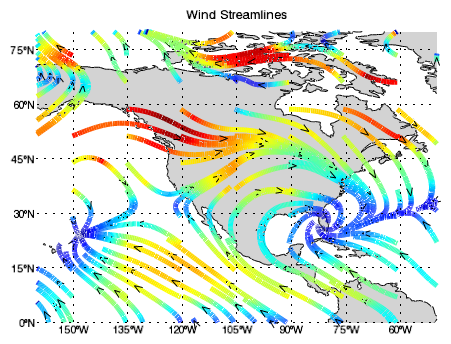The STREAMLINE function allows you to draw streamlines in a graphic. The following example displays streamlines of global wind vector data over a global map with continental outlines.The code shown below creates the graphic shown above. You can copy the entire block and paste it into the IDL command line to run it.

`; Read the data.`
`RESTORE, FILEPATH('globalwinds.dat', SUBDIR=['examples','data'])`
` `
`; Set up the map projection, grid, and continents.`
`map = MAP('Equirectangular', POSITION=[0.1,0.1,0.9,0.9], \$`
`    LIMIT=[0,-160,80,-50], TITLE='Wind Streamlines')`
` `
`; Change some map grid properties.`
`grid = map.MAPGRID`
`grid.LINESTYLE = "dotted"`
`grid.ANTIALIAS = 0`
`grid.LABEL_POSITION = 0`
`grid.LABEL_ANGLE = 0`
`grid.FONT_SIZE=11`
` `
`cont = MAPCONTINENTS(FILL_COLOR="light gray")`
` `
`; Display the streamlines on top of the map.`
`stream = STREAMLINE(u, v, x, y, /OVERPLOT, \$`
`    STREAMLINE_STEPSIZE=0.05, \$`
`   RGB_TABLE=33, AUTO_COLOR=1, THICK=5)`
` `